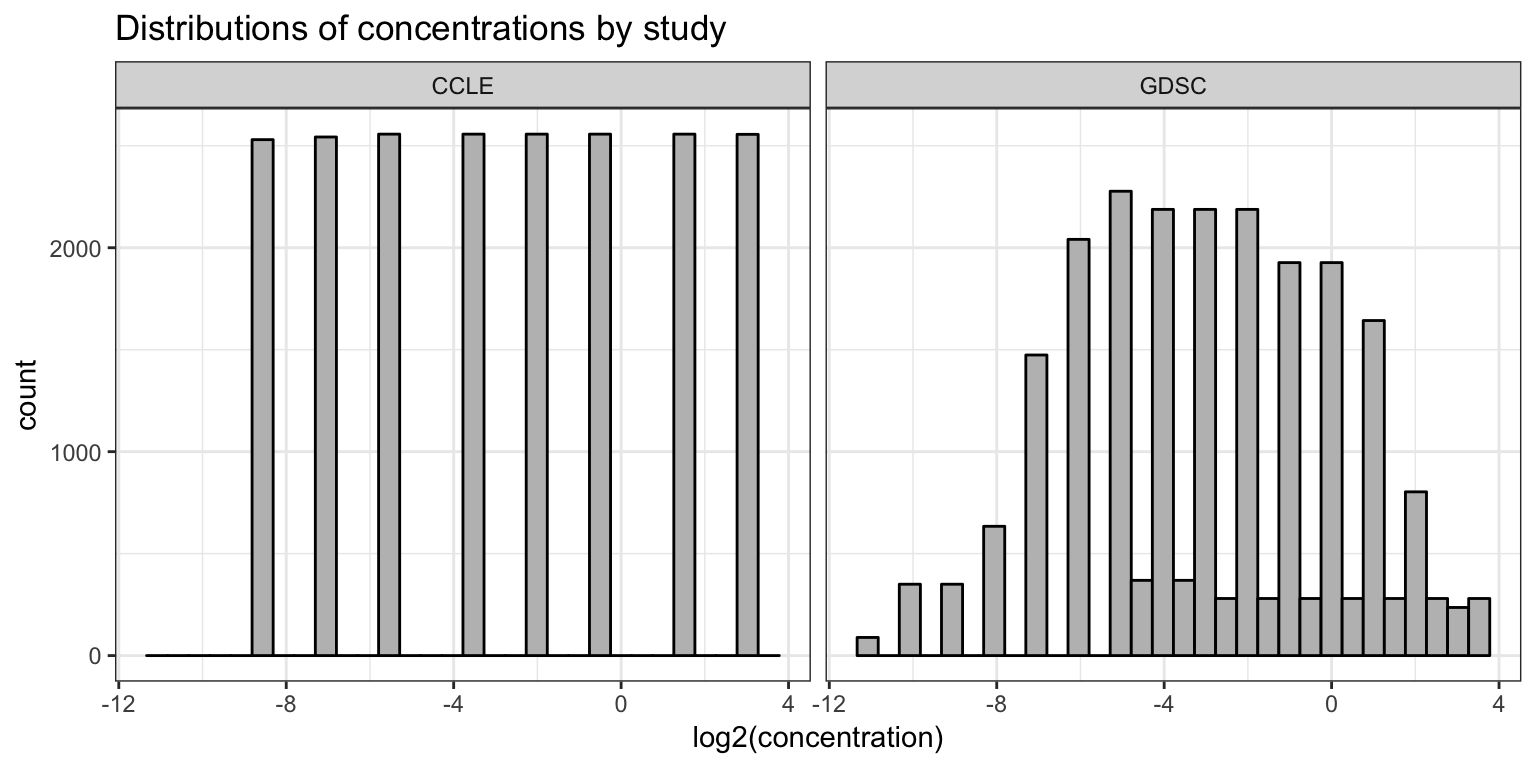## Introduction

Probably the most important step of analyzing datasets is to actually understand the data. This process is crucial to know what kind of questions we can answer with it. This tutorial has code that will help guiding you through this process with the `rawPharmacoData` dataset. Make sure you understand the experimental design of the two studies well and try to link each variable to this experimental design. Also, make sure you understand what each R command is doing. Feel free to hack the code!

When it makes sense, we include examples for answering the question using both base R and the tidyverse packages. There’s usually more than one way of doing things in R!

If you have any question about the code, ask one of the mentors. Also remember that Google search is one of the most important tools for data science.

## Setup Workspace

``library(tidyverse)``
``````## Registered S3 methods overwritten by 'ggplot2':
##   method         from
##   [.quosures     rlang
##   c.quosures     rlang
##   print.quosures rlang``````
``## ── Attaching packages ────────────────────────────────── tidyverse 1.2.1 ──``
``````## ✔ ggplot2 3.1.1     ✔ purrr   0.3.2
## ✔ tibble  2.1.2     ✔ dplyr   0.8.1
## ✔ tidyr   0.8.3     ✔ stringr 1.4.0
## ✔ readr   1.3.1     ✔ forcats 0.4.0``````
``````## ── Conflicts ───────────────────────────────────── tidyverse_conflicts() ──

There are several pre-defined themes for plotting with `ggplot2`. While the default “`theme_gray`” is nice, we will set the default to “`theme_bw`” using the `theme_set` function.

``theme_set(theme_bw())``

Let’s start by loading the `RDS` file containing the raw pharmacological data.

``pharmacoData <- readRDS(file.path("..", "data", "rawPharmacoData.rds"))``

## Exploratory Analysis

We can take a quick peek at the data using the `head` and `str` functions. What kind of variables are in the data? Are these variables numerical and/or categorical? What does each column represent?

``head(pharmacoData)``
``````##   cellLine   drug doseID concentration viability study
## 1    22RV1 17-AAG doses1        0.0025    94.100  CCLE
## 2    22RV1 17-AAG doses2        0.0080    86.000  CCLE
## 3    22RV1 17-AAG doses3        0.0250    99.932  CCLE
## 4    22RV1 17-AAG doses4        0.0800    85.000  CCLE
## 5    22RV1 17-AAG doses5        0.2500    62.000  CCLE
## 6    22RV1 17-AAG doses6        0.8000    29.000  CCLE``````
``str(pharmacoData)``
``````## 'data.frame':    43427 obs. of  6 variables:
##  \$ cellLine     : chr  "22RV1" "22RV1" "22RV1" "22RV1" ...
##  \$ drug         : chr  "17-AAG" "17-AAG" "17-AAG" "17-AAG" ...
##  \$ doseID       : chr  "doses1" "doses2" "doses3" "doses4" ...
##  \$ concentration: num  0.0025 0.008 0.025 0.08 0.25 0.8 2.53 8 0.0025 0.008 ...
##  \$ viability    : num  94.1 86 99.9 85 62 ...
##  \$ study        : chr  "CCLE" "CCLE" "CCLE" "CCLE" ...``````

Next, we can count the number of drugs and cell lines in the dataset.

``````## using base R
length(unique(pharmacoData\$cellLine))``````
``##  288``
``length(unique(pharmacoData\$drug))``
``##  15``
``````## with the tidyverse
pharmacoData %>%
summarize(nCellLines = n_distinct(cellLine),
nDrugs     = n_distinct(drug))``````
``````##   nCellLines nDrugs
## 1        288     15``````

Let’s also try something a little more complex. We can also count the number of unique drug concentrations in each study separately.

``````## with base R
tapply(pharmacoData\$concentration, pharmacoData\$study,
function(x) { length(unique(x)) })``````
``````## CCLE GDSC
##    8   32``````
``````## with the tidyverse
pharmacoData %>%
group_by(study) %>%
summarize(n = n_distinct(concentration))``````
``````## # A tibble: 2 x 2
##   study     n
##   <chr> <int>
## 1 CCLE      8
## 2 GDSC     32``````

One of the first things data scientists do when digging into new data is to explore their distributions. Histograms visualize the data distributions and can also point us towards statistical models to use. The code below transforms the concentration values to the logarithmic scale and plots a histogram separately for each study.

``````pharmacoData %>%
ggplot(aes(x = log2(concentration))) +
geom_histogram(fill = "gray", color = "black") +
facet_wrap(~ study) +
ggtitle("Distributions of concentrations by study")``````
``## `stat_bin()` using `bins = 30`. Pick better value with `binwidth`.``Based on these plots, which study would you say has the most consistent experimental protocol?

Viability scores are the percentage of cells that survive upon exposure to a certain drug. Below, we will explore the range of the data and calculate how many data points are below 0 and above 100.

``````## with base R
range(pharmacoData\$viability)``````
``##  -20.0000 319.4919``
``sum(pharmacoData\$viability < 0)``
``##  23``
``sum(pharmacoData\$viability > 100)``
``##  15778``
``````## with the tidyverse
pharmacoData %>%
summarize(min_viability = min(viability),
max_viability = max(viability),
n_too_small   = sum(viability < 0),
n_too_big     = sum(viability > 100))``````
``````##   min_viability max_viability n_too_small n_too_big
## 1           -20      319.4919          23     15778``````

We can also compare the distribution of viability scores between the two studies using density plots.

``````pharmacoData %>%
ggplot(aes(x = viability, group = study, fill = study, color = study)) +
geom_density(alpha = 1/4) +
xlim(0, 170) +
ggtitle("Distributions of viability scores by study")``````